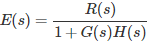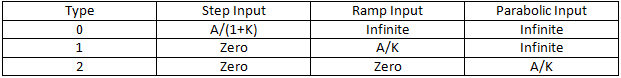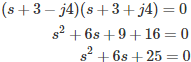# MCQs on Control Systems

##### Page 18 of 23. Go to page 1 2 3 4 5 6 7 8 9 10 11 12 13 14 15 16 17 18 19 20 21 22 23
01․ Given the Laplace transform of f(t) = F(s), the Laplace transform of (f(t)e-at) is equal to
F(s)/(s+a)
F(s+a)
e-as*F(s)
eas*F(s)

IF Laplace transform of f(t) = F(s), then the Laplace transform of (f(t)e-at) is equal to F(s+a).

02․ Consider the function F(s) = ω/(s²+ω²). Where F(s) = Laplace transform of f(t). The final value of f(t) is equal to
zero
infinite
finite constant
a value between -1 and +1

Given function F(s) = ω/(s²+ω²) By taking inverse Laplace transforms, f(t) = sinωt Therefore, the final value for sinωt is equals to a value between -1 and +1.

03․ What will be the type of the system, if the steady state performance of control system yields a non zero finite value of the velocity error constant?
type - 0
type - 1
type - 2
type - 3

Error equationApplying final value theorem, Steady state error = Lts→0 S E(s) Steady state error for different types and different inputs as follows:04․ A transfer function has a zero at s = -1 and poles at s = -1 ± j1. The multiplier being unity, if the input is step function, the steady state response is given by
0.5∠10°
0.5∠0°
1∠0°
2∠90°

Transfer function = (s+1)/((s+1-j)(s+1+j)) C(s)/R(s) = (s+1)/((s+1)²+1) Given R(s) = 1/s Steady state response C(s) s→0 = s*R(s)*transfer function = (s+1)/((s+1)²+1) Steady state response C(s) s→0 = 0.5∠0°

05․ The transfer function of the system described by d²/dt²(y(t)) + 3d/dt(y(t)) + 2y(t) = 5u(t) with u(t) as input and y(t) as output is
5/(s²+3s+2)
5/s(s²+3s+2)
(s²+3s+2)/5
s(s²+3s+2)/5

Given that d²/dt²(y(t)) + 3d/dt(y(t)) + 2y(t) = 5u(t) By taking Laplace transforms, (s²+3s+2)Y(s) = 5 U(s) Transfer function = 5/(s²+3s+2)

06․ Transfer function of a control system depends on
initial conditions of input and output.
system parameters alone.
nature of output.
nature of input.

While deriving the transfer function of a linear element only initial conditions are assumed to be zero, loading or input cannot be assumed to be zero. The transfer function is defined as the ratio of Laplace transform of the output to the Laplace transform of the input with zero initial conditions.

07․ The poles of the second order system are at -3 ± j4. The damping ratio of the system is
3/4
3/7
3/5
4/5

The characteristic equation isTherefore, Natural frequency ωn = 5 rad/s. Damping ratio = 3/5.

08․ The characteristic equation has the following roots for over damped stable system?
-2,-2
-2±j4
-2,-4
-2,2

1. For over damped system, the roots are real and unequal. 2. For under damped system, the roots are complex and conjugate. 3. For critically damped system, the roots are real and equal. 4. For un damped system, the roots are imaginary.

09․ The transfer function of a linear time invariant system is given as G(s) = 10/(s² + 10s + 10). The steady state value of the output of the system for step input applied at time instant t=2 sec will be
0
1
infinity
undefined

Transfer function G(s) = 10/(s² + 10s + 10) Input = u(t-2) L(u(t-2)) = 1/s *e-2s Final value = Lts→0 s*C(s) = Lts→0 s * 10/(s² + 10s + 10) * 1/s *e-2s Final value = 1

10․ The error of a system to a ramp input is 1/5, if the type of the system is decreased by one, then the error of unit step input is
0.2
0.167
5
6/5

The error of a system to a ramp input = 1/kv = 1/5 Kv = 5 When type of system is decreased to type zero, 'kv' of type one system becomes 'kp' of type zero system. Therefore, kp = 5 Error for step input = 1/(1+kp) = 1/6 = 0.167

<<<1617181920>>>